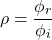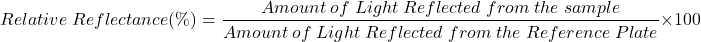# Reflectance, Reflectivity, and the Solar Reflectance Index

Contents

## What is Reflectance

Reflectance is defined as the ratio of the radiant flux reflected from the surface or body of a material (фr) to the incident radiant fluxi). Reflectance is denoted by ρ (or p).The reflectance is a ratio of radiant flux. Therefore, it is unitless. The reflectance varies as per the wavelength distribution of the incident radiation. Reflectance and transmittance are closely related concepts.

Reflectance can be categorized into two types. One is specular reflectance (ρs) and the second is diffuse reflectance (ρd).

Specular reflectance is defined as the radiant flux that is not scattering or diffusing. For example, reflection by a mirror.

Diffuse reflectance is defined as the radiant flux that is in a scattering manner. For example, reflection by cinema screen.

The total reflectance is the sum of the specular reflectance and diffuse reflectance.

## Reflectivity

Reflectivity is defined as the property of a material to reflect the light or radiation. It is a measurement of reflectance irrespective of the thickness of a material.

Reflectivity and Reflectance are the same for homogeneous and semi-infinite materials. But it is different for finite and layered material.

### Reflectance vs Reflectivity

Reflectance and Reflectivity both terms are distinguished with each other. The difference between Reflectance and Reflectivity is described as below statements.

When a light incident on a thin layer of material, the internal reflection effect causes the reflectance. It is varying with surface thickness. While reflectivity is a value that applies to thick reflecting objects.

Reflectivity is the limit value of reflectance when the sample is thick. It is the intrinsic reflection of the surface.

Reflectance is a fraction of electromagnetic power reflected form material or surface. Reflectivity is a property of a material.

## Reflection Measurement

When a light incident on a sample, it will reflect from the sample. Reflected light consists of specular reflected light and diffuse reflected light.

As shown in the below figure, the light incident on a sample at an angle of θ. This angle is known as the incident angle.

The specular reflected light is the light reflected from the shiny surface of a sample and diffuse reflected light is the light reflected from the rough surface of the sample.

When the sample has both rough and shiny surfaces, measure both reflected light and combine them to measure the total reflected light.

Reflectance measurement measure relative or absolute reflected light.

Relative reflectance measurement calculates the proportional amount of reflected light, relative to the amount of reflected light from a reference plate. The mirror or barium sulfate plate used as a reference plate. Here, we have assumed that the reflectance of a reference plate is 100%. The equation to measure the relative reflectance is;Absolute reflectance measures the proportional amount of reflected light relative to the amount of light measured directly from a light source. Here, no reference plate is used. But reflectance measurement values are based on assuming a 100% reflectance of air. The equation to measure the absolute reflectance is;## Spectral Reflectance Curve

The reflectance is a function of the wavelength of the electromagnetic energy. Spectral reflectance or Spectral reflectance curve is a graph of Wavelength vs %Reflectance.

The spectral reflectance curve is a measure of how much energy it will reflect at a different wavelength. Spectral reflectance is different for different earth surface features.

It is a curve that provides information about reflectance of material or surface for different wavelengths throughout the visible spectrum, by determining the color of material or surface.

The below figure shows the spectral reflectance curve for different surfaces for different wavelengths.

## Solar Reflectance Index

Solar reflectance index or solar reflectivity is the capability of a material to reflect solar energy from the surface of material back to the atmosphere.

The value of the solar reflectance index varies from 0 to 1. If the material absorbs all solar energy the value of the solar reflectance index is 0 and if the material reflects all solar energy the value of the solar reflectance index is 1.

## Attenuated Total Reflectance (ATR)

Attenuated Total Reflectance (ATR) is a sampling technique useful for studying the surface of a material. This technique works on the multiple internal reflections of the beam incident on the sample.

When an infrared beam incident on a high reflective index medium to low reflective index medium, some amount of light is reflected back into the low reflective index medium. This is known as internal reflection.

If the infrared beam applied at a particular angle of incident, most of all the amount of light reflected back. This is known as total internal reflection.

Some amount of lightwave runoff from the samples and extends a small distance beyond the surface. These light waves are known as an evanescent wave.

At this point, the intensity of reflected light is reduced and this phenomenon is known as the Attenuated Total Reflectance.

## Reflectance Spectroscopy

Reflectance spectroscopy is a method of measuring properties of solids and absorption spectra. This method is very useful because this type of measurement can be made without contacting the sample.

It measures the color and intensity of reflected light. The reflected light maintains the same wavelength, in spite of different wavelengths is absorbed and reflected in different degrees.

The dynamic range of measurement is extremely large. The measurement of a single spectrum can give information on the spectral absorption coefficient over the range of several spectra.

## Reflection and Transmission Coefficients

The reflection coefficient is a ratio of the amplitude of the reflected wave to the incident wave. It describes how much amount of waves can be reflected from the surface of the material or medium.

In optics, it is used to calculate the amount of light that is reflected from the surface of the medium. In electrical engineering, it is used to calculate the amount of electromagnetic waves is reflected in the transmission lines.

The reflectance coefficient and transmission coefficient are related to each other.

The transmission coefficient describes the behavior of waves incident of surface or medium. It is an amplitude of wave transmitted through a medium to that of the incident wave.

Want To Learn Faster? 🎓
Get electrical articles delivered to your inbox every week.
No credit card required—it’s 100% free.